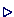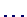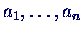## DefinitionsA new command (macro) is defined with

\newcommand{\NAME}{DEFINITION}

and used simply by \name.With the definition

\newcommand{\gi}{\Gamma_{i}}

you get the mathematical symbolsimply by \gi.This definition only works in math mode, so in text mode it has to be enclosed in \$\$. To avoid this, one can use the \ensuremath command. It ensures that its argument is in math mode, doing it explicitly, if necessary:

\newcommand{\gi}{\ensuremath{\Gamma_{i}}}A new command with parameters is defined by

\newcommand{\NAME}[NARGS]{DEFINITION}

where NARGS is the number of parameters. They are used in DEFINITION as #1, #2,After the definition

\newcommand{\veca}{\ensuremath{#1_1, \ldots, #1_n}}

the text

We consider the series \veca{a} and \veca{b}.

produces:

• We consider the seriesand.Macro definitions can appear anywhere, but are usually collected in the preamble.Macros are not only used for shortcuts, but are important to describe the logical structure of a document.Assume a document contains a lot of term definitions, which are usually displayed with bold face. The macro

\newcommand{\defi}{\textbf{#1}}

makes this logical meaning clearer than using simply \textbf. Moreover, if one decides to use italics instead and put all definitions into an index at the end, this can be done by simply changing the macro.Peter Junglas 15.5.2000